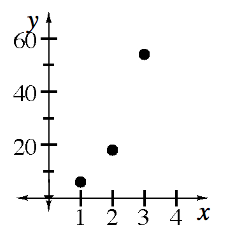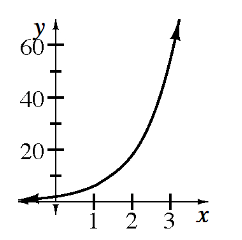### Home > CC4 > Chapter 8 > Lesson 8.1.1 > Problem8-8

8-8.

A particular sequence can be represented by $t(n) = 2(3)^n$. Homework Help ✎

1. What are $t(1)$, $t(2)$, and $t(3)$?

To find $t(0)$, substitute $0$ in for $n$ in the equation$t(n) = 2(3)^n$. So $t(0) = 2(3)^0$.

$t(1) = 6$,
$t(2) = 18$,
$t(3) = 54$

2. Graph this sequence. What is the domain?Domain: non-negative integers

3. On the same set of axes, graph the function $f(x) = 2(3)^x$ .4. How are the two graphs similar? How are they different?

How do their shapes compare?
Are they discrete or continuous?